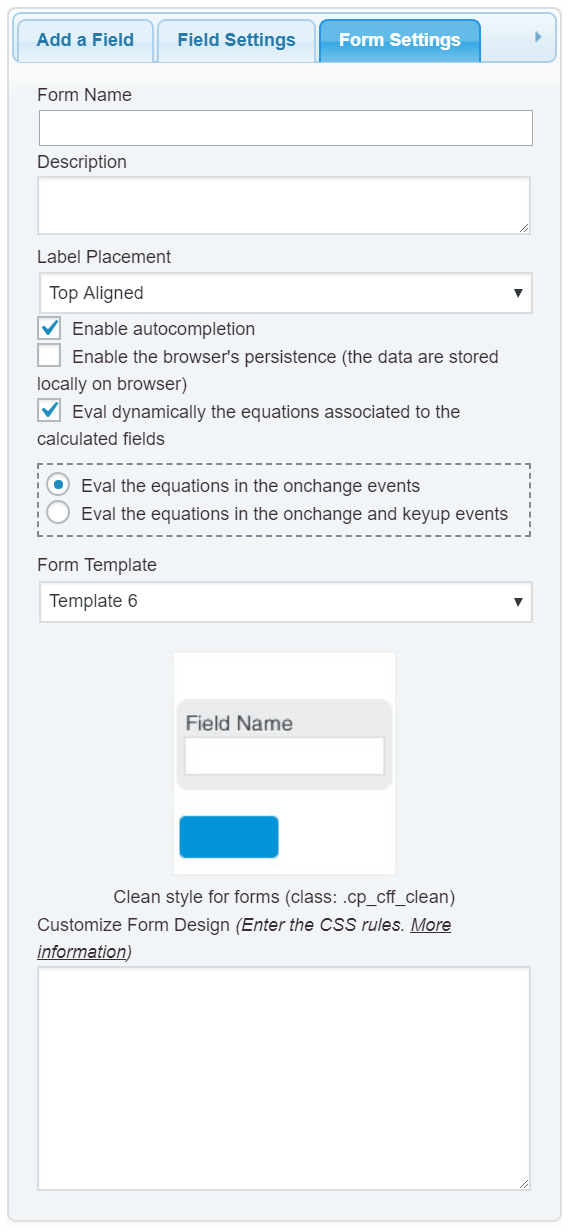Support » Plugin: Calculated Fields Form » Missing values / replace values

• Hi,

(1) let’s say I have four number fields (fieldname 1 – 4) and one calculated field (fieldname5) with the equation (fieldname1+fieldname2+fieldname3+fieldname4)/4. Possible values are 0 – 3.

What I need is a way on how to deal with missing values: Is it possible to replace specific values? If the user enters “9” for a missing value, I would like to replace this value automatically with 1.5.

So, if the user enters:
fieldname1 = 2
fieldname2 = 0
fieldname3 = 9
fieldname4 = 1
The result should be 1.125 ((2+0+1.5+1/4))

What I need is such a function globally before calculating the fields, thus
1. Enter field values
2. fire calculation button by onclick=”evaluate_equations(this);”
3. replace values
4. calculate the “calculated fields

(2) I am validations, so a user can f.e. onlye enter numbers from 0 – 2 for the number fields. If I am using a “9” as placeholder for missing values, how to handle the validations? Is there a way to define a validation rule like “all values from 0 – 2 AND 9” ?

Thanks!

Viewing 3 replies - 1 through 3 (of 3 total)
• Hello @tuempelkoenig

I’m not sure to understand exactly your description of issue.

So, I’ll try to answer you based on my understanding of your description. Assuming you want to accept only values between 0 and 2 (including both) in the number fields, you simply should enter the number 0 into the “Min” attribute in their settings, and the number 2 into the “Max” attribute. Furthermore, the values of fields that were left in blank by the user, would be considered as 1.5 in the equation.

To implement this behavior, the equation should be edited as follows:

``````
(function(){
var a = IF(fieldname1|r == '', 1.5, fieldname1),
b = IF(fieldname2|r == '', 1.5, fieldname2),
c = IF(fieldname3|r == '', 1.5, fieldname3),
d = IF(fieldname4|r == '', 1.5, fieldname4);

return IF(AND(0 <= a, a <= 2, 0 <= b, b <= 2,0 <= c, c <= 2,0 <= d, d <= 2), (a+b+c+d)/4, '');
})()
``````

Finally, if you don’t want the equations be evaluated dynamically, only after the user press a button in the form, please, follow the instructions below:

– Go to the “Form Settings” tab, and untick the checkbox: “Eval dynamically the equations associated to the calculated fields”– Insert a button field in the form, and select as the button’s type the “Calculate” option (it is a radio button in the field’s settings).

and that’s all.
Best regards.

Hi, not exactly 🙂 I’ll try to explain a bit more.

Assuming you want to accept only values between 0 and 2 (including both) in the number fields, you simply should enter the number 0 into the “Min” attribute in their settings, and the number 2 into the “Max” attribute.

This is clear to me. I want to add a new validation rule to validate for
Min value = 0 | Max value = 2 OR value = 9

Furthermore, the values of fields that were left in blank by the user, would be considered as 1.5 in the equation.

It’s more simple: I want all values of “9” replace by “1.5” BEFORE calculating the calculated fields. Perhaps something like:

\$( “input[type=’number’]” ).change(function() {
// change all input fields with value “9” to value “1!
});

Hope it’s more clear and thanks for your great support!

Hello @tuempelkoenig

The process is simple, follow the instructions in the previous message, but editing the equation as follows:

``````
(function(){
var a = fieldname1,
b = fieldname2,
c = fieldname3,
d = fieldname4;

return IF(AND(0 <= a, a <= 2, 0 <= b, b <= 2,0 <= c, c <= 2,0 <= d, d <= 2), (a+b+c+d)/4, '');
})()
``````

and follow too the instructions below:

– Assign to these number fields a custom class name to identify them, for example: my-special-number-field

Note: the class names are assigned to the fields through their attributes: “Add CSS Layout Keywords”

– And then, insert a “HTML Content” field in the form with the following piece of code as its content:

``````
<script>
jQuery(document).on('change', '.my-special-number-field input', function(){
if(this.value == 9)
{
this.value=1.5;
jQuery(this).change();
}
});
</script>
``````

and that’s all.

The values accepted by the number fields are between 0 to 2 (including both), but if the user enter the number 9, the form will transform it into 1.5 and will evaluate the equation again.

Best regards.

Viewing 3 replies - 1 through 3 (of 3 total)
• You must be logged in to reply to this topic.